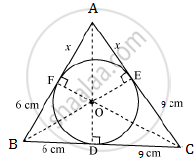# sdfsdf - Mathematics

In the below figure, a triangle ABC is drawn to circumscribe a circle of radius 3 cm, such that the segments BD and DC are respectively of lengths 6 cm and 9 cm. If the area of Δ ABC is 54 cm2, then find the lengths of sides AB and AC.#### SolutionLet the given circle touch the sides AB and AC of the triangle at points F and E respectively and let the length of the line segment AF be x.

Now, it can be observed that:
BF = BD = 6 cm (tangents from point B)
CE = CD = 9 cm (tangents from point C)
AE = AF = x (tangents from point A)

AB = AF + FB = x + 6
BC = BD + DC = 6 + 9 = 15
CA = CE + EA = 9 + x
2s = AB + BC + CA = x + 6 + 15 + 9 + x = 30 + 2x
s = 15 + x
s – a = 15 + x – 15 = x
s – b = 15 + x – (x + 9) = 6
s – c = 15 + x – (6 + x) = 9

"Area of " triangleABC =sqrt(s(s-a)(s-b)(s-c))

54=sqrt((15+x)(x)(6)(9))

54=3sqrt(6(15x+x^2))

18=sqrt(6(15x+x^2))

324=6(15x+x^2)

54=15x+x^2

x^2+15x-54=0

x^2+18x-3x-54=0

x(x+18)-3(x+18)=0

(x+18)(x-3)=0

x = -18 and x = 3
As distance cannot be negative, x = 3
AC = 3 + 9 = 12
AB = AF + FB = 6 + x = 6 + 3 = 9

Concept: Triangles Examples and Solutions
Is there an error in this question or solution?
2014-2015 (March) All India Set 2

Share